Latest Banking jobs   »   Basic Quantitative Aptitude Quiz for All...

# Basic Quantitative Aptitude Quiz for All Banking Exams- 20th May

Directions (1-2): The following questions are accompanied by two statements A and B. You have to determine which statements(s) is/are sufficient/necessary to answer the questions.
(a) Both the statements taken together are necessary to answer the questions, but neither of the statements alone is sufficient to answer the question.
(b) Statement B alone is sufficient to answer the question, but statement A alone is not sufficient to answer the question.
(c) Either statement A or statement B by itself is sufficient to answer the question.
(d) Statement A alone is sufficient to answer the question, but statement B alone is not sufficient to answer the questions.
(e) Statements A and B taken together are not sufficient to answer the question.

Q1. Find the value of ‘R’.
(A) Satish invested Rs. 10,000 at R% p.a. at C.I. for 2 years and Rs. 8,000 at R% p.a. at S.I. for 4 years. He got same amount from both scheme.
(B) Ratio between principal invested at R% p.a. at CI to interest earned after 2 years is 25 : 11.

Q2. Find the volume of given cylinder if height of cylinder is 14 cm ?
(A) Total surface area of cylinder is 924 cm².
(B) Total surface area of cylinder is 50% more than of curved surface area of cylinder.

Directions (3-5): Each of the following questions is provided with 2 statements i.e. Statement I & Statement II. You have to study them and find which statement (s) is/are necessary to answer the question as per the instruction set given below.
(a) Only Statement I is necessary
(b) Either Statement I or Statement II is sufficient to answer
(c) Both Statement I & Statement II are necessary to answer
(d) Only Statement II is necessary
(e) Neither Statement I nor Statement II is sufficient to answer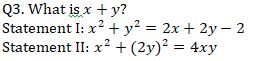Q4. How many members are there in the group initially?
Statement I: average age of group is 20 year.
Statement II: the average age remains same when a new person 20 years old joins the group.

Q5. What is present monthly salary of Gulati?
Statement I: Gulati spends Rs. 4000 on rent and clothes which is 20% of his salary.
Statement II: Gulati spends Rs. 2000 on rent and saves Rs. 1500 every month.

Directions (6-10): Each of the following questions is provided with 2 statements i.e. Statement I & Statement II. You have to study them and find which statement (s) is/are necessary to answer the question as per the instruction set given below.
(a) Only Statement I is sufficient
(b) Only Statement II is sufficient
(c) Neither Statement I nor Statement II is sufficient
(d) Either Statement I or Statement II is sufficient
(e) Both Statement I & Statement II together is sufficient

Q6. What is speed of car?
Statement I: Car can cover a distance of 100 km in 2 hour.
Statement II: Speed of bike is 20 kmph which is 40% of speed of car.

Q7. What is length of platform?
Statement I: a train covers the platform in 20 sec.
Statement II: the length of train is 50% of length of platform.

Q8. What is ratio of boys to girls in the class?
Statement I: total students in class are 100.
Statement II: boys are 20% more than girls.

Q9. What is present salary of Sanjay?
Statement I: Sanjay saves Rs. 500 which is 10% of his salary.
Statement II: Sanjay spends Rs. 2000 more than he saves.

Q10. What is present population?
Statement I: the population last year was 1000.
Statement II: population increases by 10% every year.

Directions (11-15): Each of the following questions is provided with 2 statements i.e. Statement I & Statement II. You have to study them and find which statement (s) is/are necessary to answer the question as per the instruction set given below.
(a) Only Statement I is necessary
(b) Only Statement II is necessary
(c) Neither Statement I nor Statement II
(d) Either Statement I or Statement II is necessary.
(e) Both Statement I & Statement II together are necessary.

Q11. What is ratio of a to b?
Statement I: 50% of a is 25% of b.
Statement II: 30% of a is 75% of b.

Q12. How many people (male + female) are there in aeroplane?
Statement I: There are 45 females in aeroplane.
Statement II: 36% of total passengers are females.

Q13. What is age of P?
Statement I: average age of P and R is 12 years.
Statement II: R is younger than P.

Q14. What is the area of floor?
Statement I: Flooring the hall at the rate Rs. 100/sq.m. is Rs. 10000.
Statement II: painting cost of floor is Rs. 25 per sq.m.

Q15. What is area of right angle triangle (having integral sides)?
Statement I: The perimeter of right angle triangle is equal to perimeter of square having side 5 cm.
Statement II: length of hypotenuse is 5 cm.

Practice More Questions of Quantitative Aptitude for Competitive Exams:

Solutions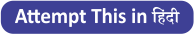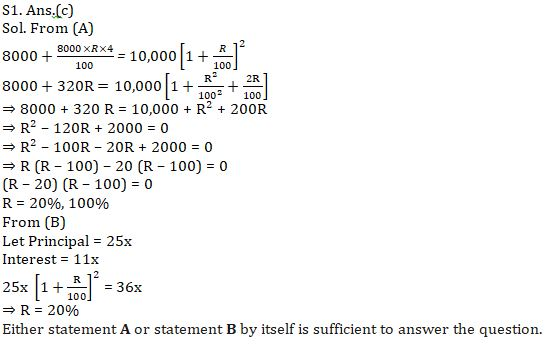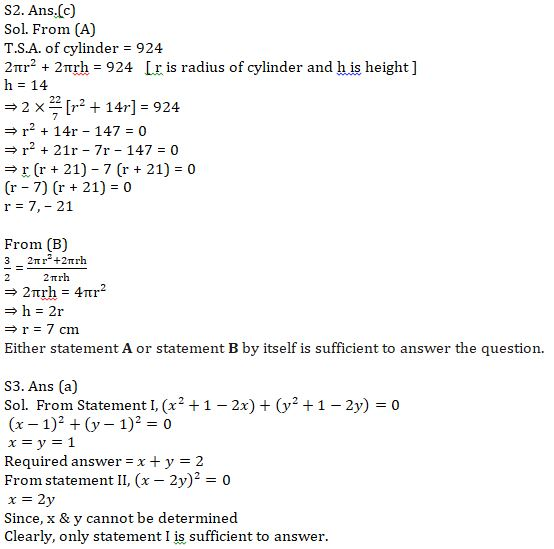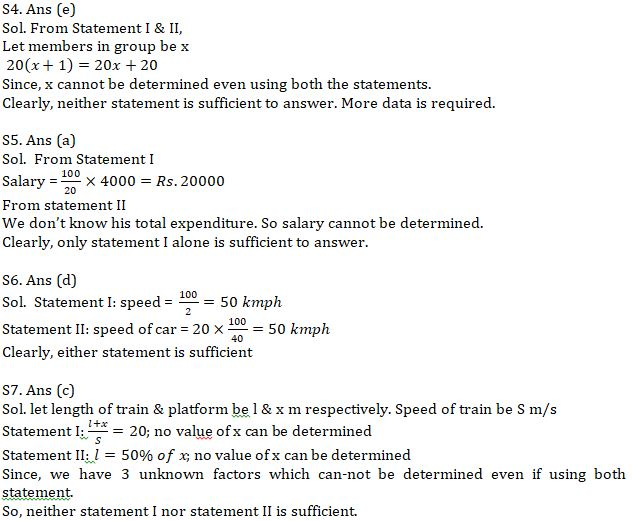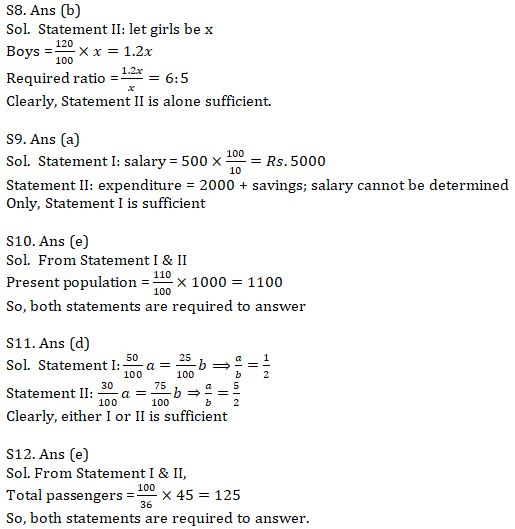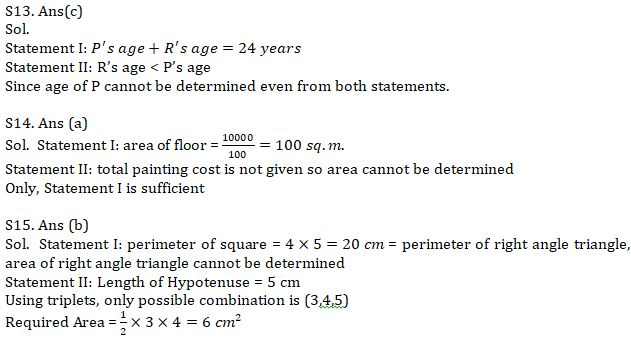#### Congratulations!Incorrect details? Fill the form again here

•Reasoning Ability Quiz For IBPS RRB PO C...
•Quantitative Aptitude Quiz For IBPS RRB ...
•Important Current Affairs Quiz for Bank ...
•Reasoning Ability Quiz For IBPS Clerk Pr...
•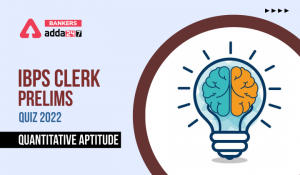Quantitative Aptitude Quiz For IBPS Cler...
•English Quizzes For IBPS Clerk Prelims 2...